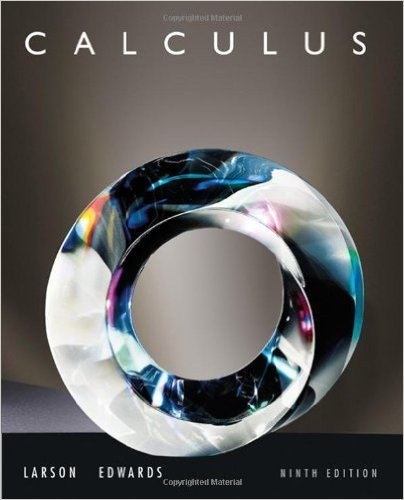×
×

# Solutions for Chapter 13.5: Chain Rules for Functions of Several Variables## Full solutions for Calculus | 9th Edition

ISBN: 9780547167022Solutions for Chapter 13.5: Chain Rules for Functions of Several Variables

Solutions for Chapter 13.5
4 5 0 293 Reviews
17
2
##### ISBN: 9780547167022

This textbook survival guide was created for the textbook: Calculus , edition: 9. This expansive textbook survival guide covers the following chapters and their solutions. Chapter 13.5: Chain Rules for Functions of Several Variables includes 65 full step-by-step solutions. Calculus was written by and is associated to the ISBN: 9780547167022. Since 65 problems in chapter 13.5: Chain Rules for Functions of Several Variables have been answered, more than 64323 students have viewed full step-by-step solutions from this chapter.

Key Calculus Terms and definitions covered in this textbook
• Arc length formula

The length of an arc in a circle of radius r intercepted by a central angle of u radians is s = r u.

• Arcsine function

See Inverse sine function.

• Chord of a conic

A line segment with endpoints on the conic

• Domain of validity of an identity

The set of values of the variable for which both sides of the identity are defined

• Double-blind experiment

A blind experiment in which the researcher gathering data from the subjects is not told which subjects have received which treatment

• Equivalent systems of equations

Systems of equations that have the same solution.

• Exponent

See nth power of a.

• Law of cosines

a2 = b2 + c2 - 2bc cos A, b2 = a2 + c2 - 2ac cos B, c2 = a2 + b2 - 2ab cos C

• Linear regression line

The line for which the sum of the squares of the residuals is the smallest possible

• Logarithmic re-expression of data

Transformation of a data set involving the natural logarithm: exponential regression, natural logarithmic regression, power regression

• Lower bound test for real zeros

A test for finding a lower bound for the real zeros of a polynomial

• NDER ƒ(a)

See Numerical derivative of ƒ at x = a.

• Opposite

See Additive inverse of a real number and Additive inverse of a complex number.

The graph in three dimensions of a seconddegree equation in three variables.

• Real zeros

Zeros of a function that are real numbers.

• Root of a number

See Principal nth root.

• Scatter plot

A plot of all the ordered pairs of a two-variable data set on a coordinate plane.

• Standard form of a polar equation of a conic

r = ke 1 e cos ? or r = ke 1 e sin ? ,

• Third quartile

See Quartile.

• Viewing window

The rectangular portion of the coordinate plane specified by the dimensions [Xmin, Xmax] by [Ymin, Ymax].

×